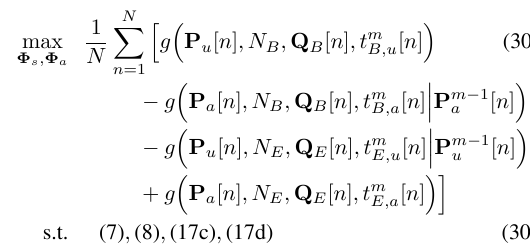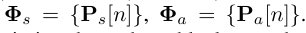# How to express the complex objective function in cvx?

The objective function is related to summation, and the problem is as follows:where,

.and ‘m’ represents the m-th iteration. Similarly, ‘m-1’ denots the (m-1)-th iteration.

In the objective function , N is large, i.e., 100~1000. In addition, I_L is an unity matrix (L=2), N_B=5, N_E=3. Q_B is a matrix with size LLN, and Q_E is also a matrix with size LLN. P_u and P_a are diagonal matrices with size LLN.

I think it is very difficult for me to express the objective function when N is large, and I don’t think it could be expressed with for-loop if N is very large.